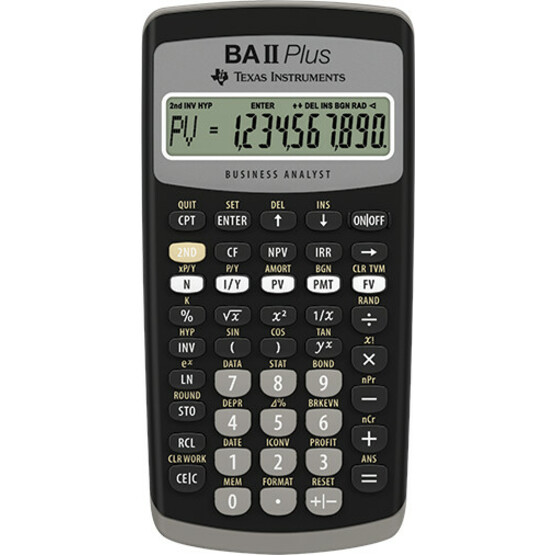Navigate: Home / Hardware / Calculators / Financial Calculators / Texas Instruments BA-II Plus Financial Calculator

# Texas Instruments BA-II Plus Financial Calculator

Solves time-value-of-money calculations such as annuities, mortgages, leases, savings, and more!
*Price: \$39.95
 SKU: BAIIPLUS-X Mfr Part #: Other: BA-II-PLUS Requires Academic ID: No
Limit one sharing discount per purchase.
 This esy-to-use Financial Calculator solves time-value-of-money calculations such as annuities, mortgages, leases, savings, and more. Generate amortization schedules. Compute NPV, IIR, break-even calculations, interest conversions, delta percent, profit and loss, cost, selling price, margin and markup. Performs cash-flow analysis for up to 24 uneven cash flows with up to four-digit frequencies.The calculator offers depreciation schedules; two day-count methods (actual or 30/360) to calculate bond price or yield to maturity or to call; list-based one-variable and two-variable statistics with four regression options: linear, logarithmic, exponential and power. Math functions include trigonometric calculations, natural logarithms, and powers. Design also features 10-digit display and 10 user memories. Automatic power-down conserves power.Calculator includes a protective slide case and one lithium 2032 battery. Functions include: NPV IIR Break-even calculation Interest conversion Delta percent Profit and loss Cost Selling price Margin Markup Linear Logarithmic Exponential Trigonometric calculation Natural logarithm